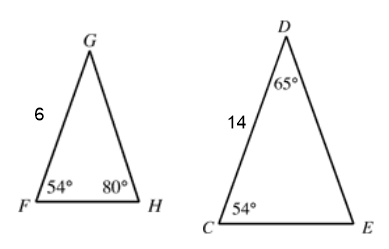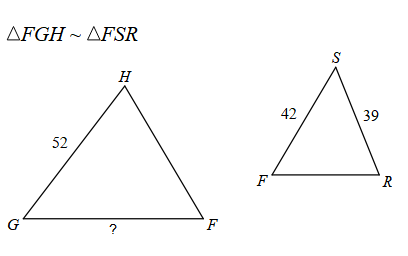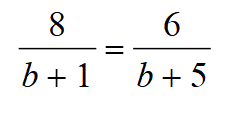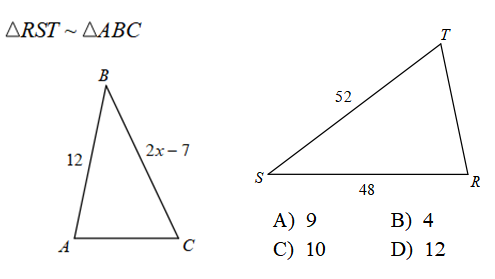GeoB similar Maintenance 3
Last Name *
Identify the relationship between these two triangles *
1 pointFind the missing side *
1 pointsolve the proportion *
1 pointIf a 3 foot yard stick casts a shadow of 21 feet. Find the height of a building that casts a shadow of 168 feet. *
1 point
Use the similar statement to setup proportions and solve for x. *
1 pointA small triangle has a perimeter of 36. The ratio to a bigger triangle is 5:2. What is the perimeter of the bigger triangle? *
1 point
period *